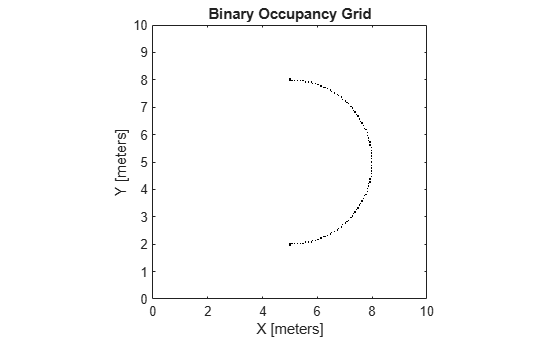Main Content

# getOccupancy

Get occupancy value of locations

## Syntax

``occVal = getOccupancy(map,xy)``
``occVal = getOccupancy(map,xy,"local")``
``occVal = getOccupancy(map,ij,"grid")``
``[occVal,validPts] = getOccupancy(___)``
``occMatrix = getOccupancy(map)``
``occMatrix = getOccupancy(map,bottomLeft,matSize)``
``occMatrix = getOccupancy(map,bottomLeft,matSize,"local")``
``occMatrix = getOccupancy(map,topLeft,matSize,"grid")``

## Description

example

````occVal = getOccupancy(map,xy)` returns an array of occupancy values at the `xy` locations in the world frame. Unknown locations, including outside the map, return `map.DefaultValue`.```

example

````occVal = getOccupancy(map,xy,"local")` returns an array of occupancy values at the `xy` locations in the local frame.```

example

````occVal = getOccupancy(map,ij,"grid")` specifies `ij` grid cell indices instead of `xy` locations.```
````[occVal,validPts] = getOccupancy(___)` additionally outputs an `n`-element vector of logical values indicating whether input coordinates are within the map limits.```
````occMatrix = getOccupancy(map)` returns all occupancy values in the map as a matrix.```
````occMatrix = getOccupancy(map,bottomLeft,matSize)` returns a matrix of occupancy values by specifying the bottom-left corner location in world coordinates and the matrix size in meters.```
````occMatrix = getOccupancy(map,bottomLeft,matSize,"local")` returns a matrix of occupancy values by specifying the bottom-left corner location in local coordinates and the matrix size in meters.```
````occMatrix = getOccupancy(map,topLeft,matSize,"grid")` returns a matrix of occupancy values by specifying the top-left cell index in grid indices and the matrix size.```

## Examples

collapse all

Create an empty binary occupancy grid map.

`map = binaryOccupancyMap(10,10,20);`

Input pose of the vehicle, ranges, angles, and the maximum range of the laser scan.

```pose = [5,5,0]; ranges = 3*ones(100,1); angles = linspace(-pi/2,pi/2,100); maxrange = 20;```

Create a `lidarScan` object with the specified ranges and angles.

`scan = lidarScan(ranges,angles);`

Insert the laser scan data into the occupancy map.

`insertRay(map,pose,scan,maxrange);`

Show the map to see the results of inserting the laser scan.

`show(map)`Check the occupancy of the spot directly in front of the vehicle.

`getOccupancy(map,[8 5])`
```ans = logical 1 ```

Access occupancy values and check their occupancy status based on the occupied and free thresholds of the `occupancyMap` object.

Create a matrix and populate it with values. Use this matrix to create an occupancy map.

```p = zeros(20,20); p(11:20,11:20) = ones(10,10); map = binaryOccupancyMap(p,10); show(map)```Get the occupancy of different locations and check their occupancy statuses. The occupancy status returns 0 for free space and 1 for occupied space. Unknown values return –1.

```pocc = getOccupancy(map,[1.5 1]); occupied = checkOccupancy(map,[1.5 1]); pocc2 = getOccupancy(map,[5 5],'grid');```

## Input Arguments

collapse all

Map representation, specified as a `binaryOccupancyMap` object. This object represents the environment of the vehicle.

Coordinates in the map, specified as an n-by-2 matrix of ```[x y]``` pairs, where n is the number of coordinates. Coordinates can be world or local coordinates depending on the syntax.

Data Types: `double`

Grid locations in the map, specified as an n-by-2 matrix of ```[i j]``` pairs, where n is the number of locations. Grid locations are given as `[row col]`.

Data Types: `double`

Location of bottom left corner of output matrix in world or local coordinates, specified as a two-element vector, `[xCoord yCoord]`. Location is in world or local coordinates based on syntax.

Data Types: `double`

Output matrix size, specified as a two-element vector, ```[xLength yLength]``` or `[gridRow gridCol]`. The size is in world coordinates, local coordinates, or grid indices based on syntax.

Data Types: `double`

Location of top left corner of grid, specified as a two-element vector, `[iCoord jCoord]`.

Data Types: `double`

## Output Arguments

collapse all

Occupancy values, returned as an n-by-1 column vector equal in length to `xy` or `ij`. Occupancy values can be obstacle free (0) or occupied (1).

Valid map locations, returned as an n-by-1 column vector equal in length to `xy` or `ij`. Locations inside the map return a value of 1. Locations outside the map limits return a value of 0.

Matrix of occupancy values, returned as matrix with size equal to `matSize` or the size of `map`.

## See Also

### Topics

Introduced in R2015a